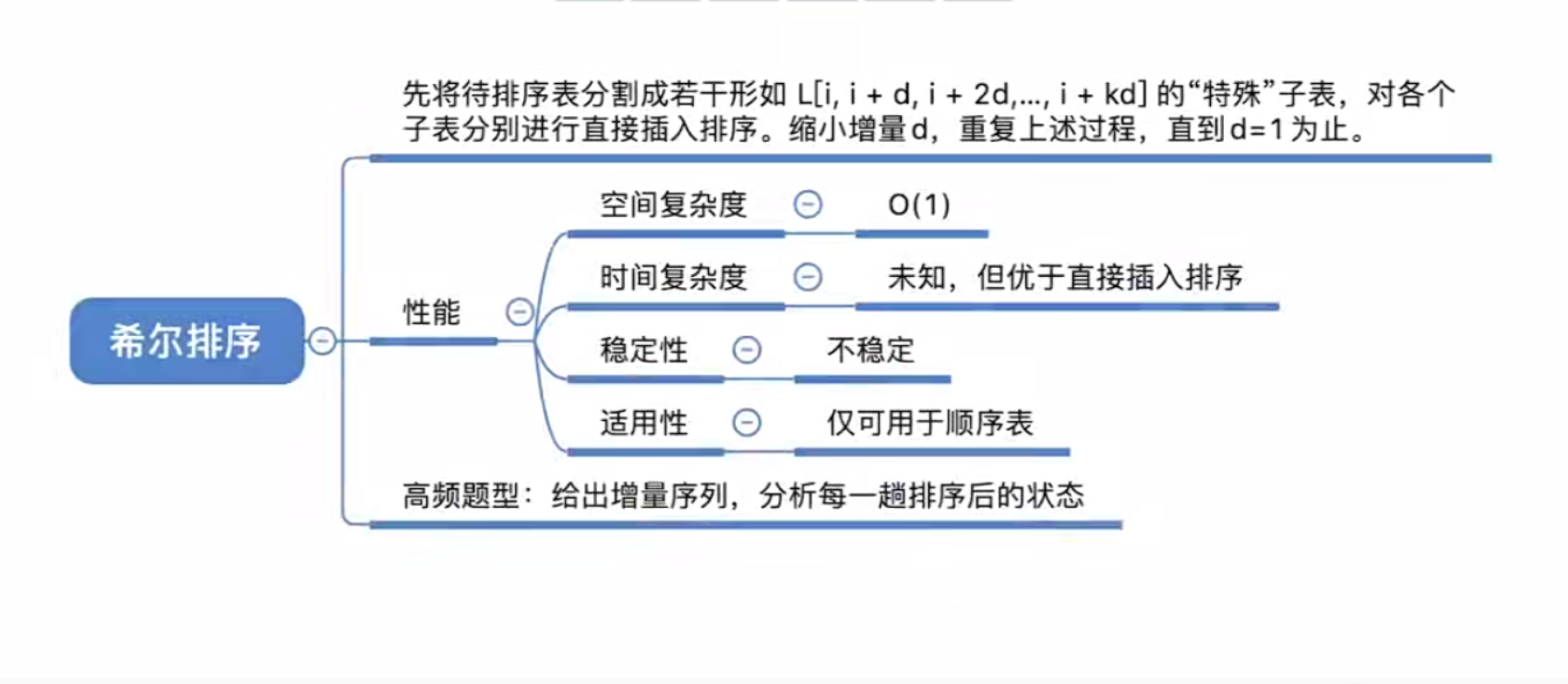# Hill sort

Recently, I have been studying data structures in a comprehensive way, and the commonly used algorithm records: Hill sorting. The basic idea is to select an increment \$d<n\$, group elements by this increment (all elements that are separated by \$d\$ are a group), and then Insertion sorting is performed in each subsequence, and the increment \$d'(d'<d)\$ is reduced after completion, and the operation is repeated until the increment \$d = 1\$. At this time, it becomes a standard insertion sort, but At this point, most of the elements are already in order, and only a few operations, or even no operations, are required to complete the sorting. The sorting algorithm is unstable sorting.

Hill’s sorting is still quite convoluted, and you need to read more and draw more.

Worst time complexity: \$O(n^2)\$
\$n\$ is reachable in a range: \$O(n^{1.3})\$
The exact time complexity cannot be proved mathematically at present.

` `#include <iostream> using namespace std; void shellSort(int arr[], int n); int main() { int arr[] = {-1, 5, 7, 12, 6, 2, 0, 8, 15, 1, 11}; int length = (int)(sizeof(arr) / sizeof(int)); //数组长度shellSort(arr, length); for (int i = 1; i < length; i++) cout << arr[i] << " "; return 0; } void shellSort(int arr[], int n) { int d, i, j; //arr为暂存单元for (d = n / 2; d > 0; d /= 2) //d为步长{ for (i = d + 1; i <= n; i++) //从子表中第二个元素开始if(arr[i] < arr[i - d]) //小于子序列前一项{ arr = arr[i]; //暂存待插入元素for (j = i - d; j > 0 && arr < arr[j]; j -= d) arr[j + d] = arr[j]; //向后移动，为待插入元素腾出空位arr[j + d] = arr; //插入暂存元素} } }``